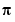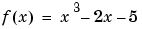MATLAB Function Referencefminbnd

Minimize a function of one variable on a fixed interval

Syntax

• ```x = fminbnd(fun,x1,x2)
x = fminbnd(fun,x1,x2,options)
x = fminbnd(fun,x1,x2,options,P1,P2,...)
[x,fval] = fminbnd(...)
[x,fval,exitflag] = fminbnd(...)
[x,fval,exitflag,output] = fminbnd(...)
```

Description

`fminbnd` finds the minimum of a function of one variable within a fixed interval.

```x = fminbnd(fun,x1,x2) ``` returns a value `x` that is a local minimizer of the function that is described in `fun` in the interval `x1 <= x <= x2`.

```x = fminbnd(fun,x1,x2,options) ``` minimizes with the optimization parameters specified in the structure `options`. You can define these parameters using the `optimset` function. `fminbnd` uses these `options` structure fields:

 `Display` Level of display. `'off'` displays no output; `'iter'` displays output at each iteration; `'final`' displays just the final output; `'notify'` (default) dislays output only if the function does not converge. `MaxFunEvals` Maximum number of function evaluations allowed. `MaxIter` Maximum number of iterations allowed. `TolX` Termination tolerance on `x.`

```x = fminbnd(fun,x1,x2,options,P1,P2,...) ``` provides for additional arguments, `P1`, `P2`, etc., which are passed to the objective function, `fun(x,P1,P2,...)`. Use `options=[]` as a placeholder if no options are set.

```[x,fval] = fminbnd(...) ``` returns the value of the objective function computed in `fun` at `x`.

```[x,fval,exitflag] = fminbnd(...) ``` returns a value `exitflag` that describes the exit condition of `fminbnd`:

 `>0` Indicates that the function converged to a solution `x.` ` 0` Indicates that the maximum number of function evaluations was exceeded. `<0` Indicates that the function did not converge to a solution.

```[x,fval,exitflag,output] = fminbnd(...) ``` returns a structure `output` that contains information about the optimization:

 `output.algorithm` The algorithm used `output.funcCount` The number of function evaluations `output.iterations` The number of iterations taken

Arguments

`fun` is the function to be minimized. `fun` accepts a scalar `x` and returns a scalar `f`, the objective function evaluated at `x`. The function `fun` can be specified as a function handle.

• ```x = fminbnd(@myfun,x0)
```

where `myfun` is a MATLAB function such as

• ```function f = myfun(x)
f = ...         % Compute function value at x.
```

`fun` can also be an inline object.

• ```x = fminbnd(inline('sin(x*x)'),x0);
```

Other arguments are described in the syntax descriptions above.

Examples

`x = fminbnd(@cos,3,4)` computesto a few decimal places and gives a message on termination.

• ```[x,fval,exitflag] =
fminbnd(@cos,3,4,optimset('TolX',1e-12,'Display','off'))
```

computesto about 12 decimal places, suppresses output, returns the function value at `x`, and returns an `exitflag` of 1.

The argument `fun` can also be an inline function. To find the minimum of the functionon the interval `(0,2)`, create an inline object `f`

• ```f = inline('x.^3`-2`*`x`-5');
```

Then invoke `fminbnd` with

• ```x = fminbnd(f`,` 0`,` 2)
```

The result is

• ```x =
0.8165
```

The value of the function at the minimum is

• ```y = f(x)

y =
-6.0887
```

Algorithm

The algorithm is based on Golden Section search and parabolic interpolation. A Fortran program implementing the same algorithm is given in .

Limitations

The function to be minimized must be continuous. `fminbnd` may only give local solutions.

`fminbnd` often exhibits slow convergence when the solution is on a boundary of the interval.

`fminbnd` only handles real variables.

`fminsearch`, `fzero`, `optimset`, `function_handle` (`@`), `inline`fmin fmins